# RD Sharma Solutions for Class 11 Chapter 19 - Arithmetic Progressions Exercise 19.6

Exercise 19.6 deals with problems related to the insertion of arithmetic means, which also contain, insertion of single arithmetic mean between two numbers. Students can easily download the pdf and refer to it while practising the textbook problems. Important formulas that are used in solving the problems are mentioned in order to help students remember the formulas to ace the exam. The solutions pdf is an important study material for students who find difficulty in solving problems. To understand the concepts, students can access RD Sharma Class 11 Maths Solutions free pdf using the links, which are available here.

## Download the pdf of RD Sharma Solutions for Class 11 Maths Exercise 19.6 Chapter 19 – Arithmetic Progressions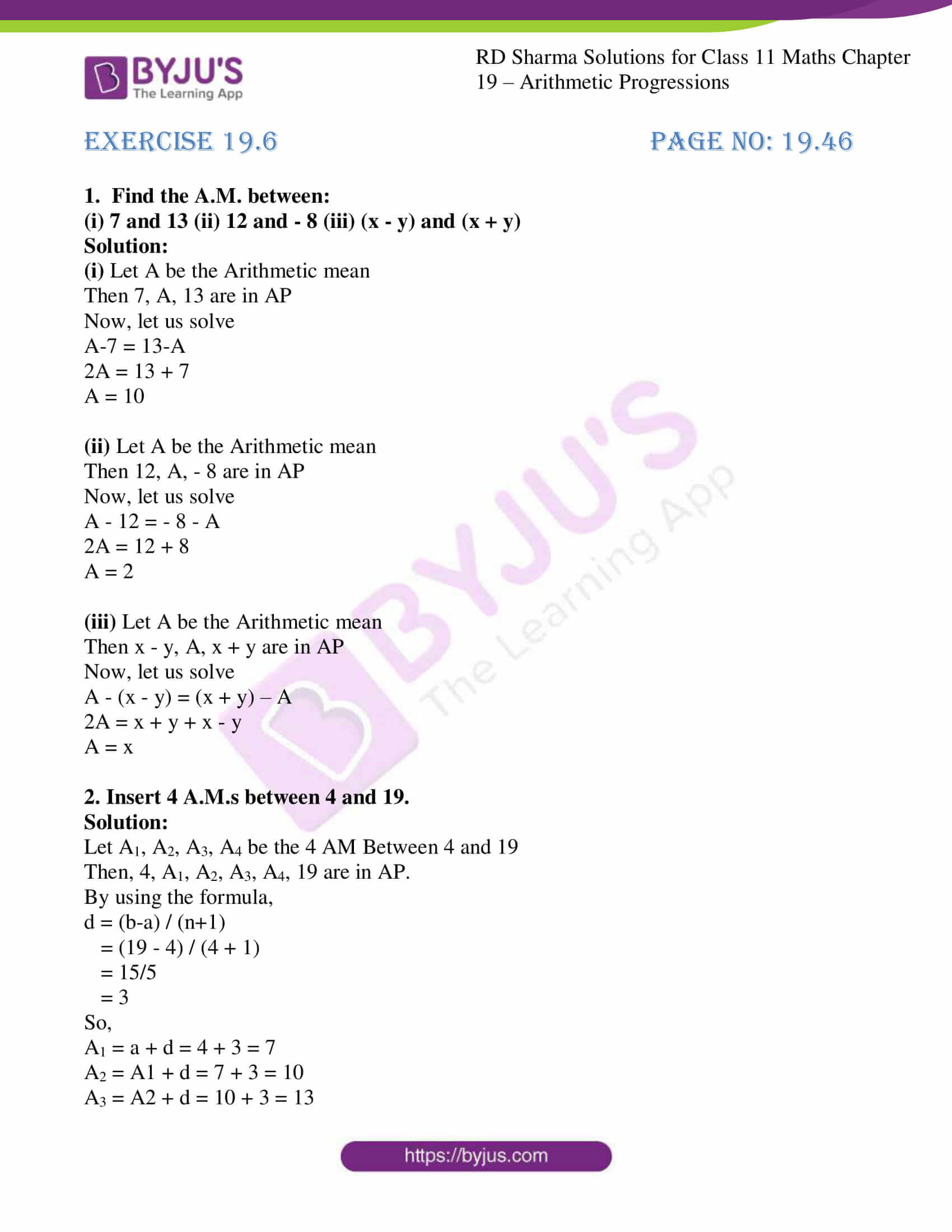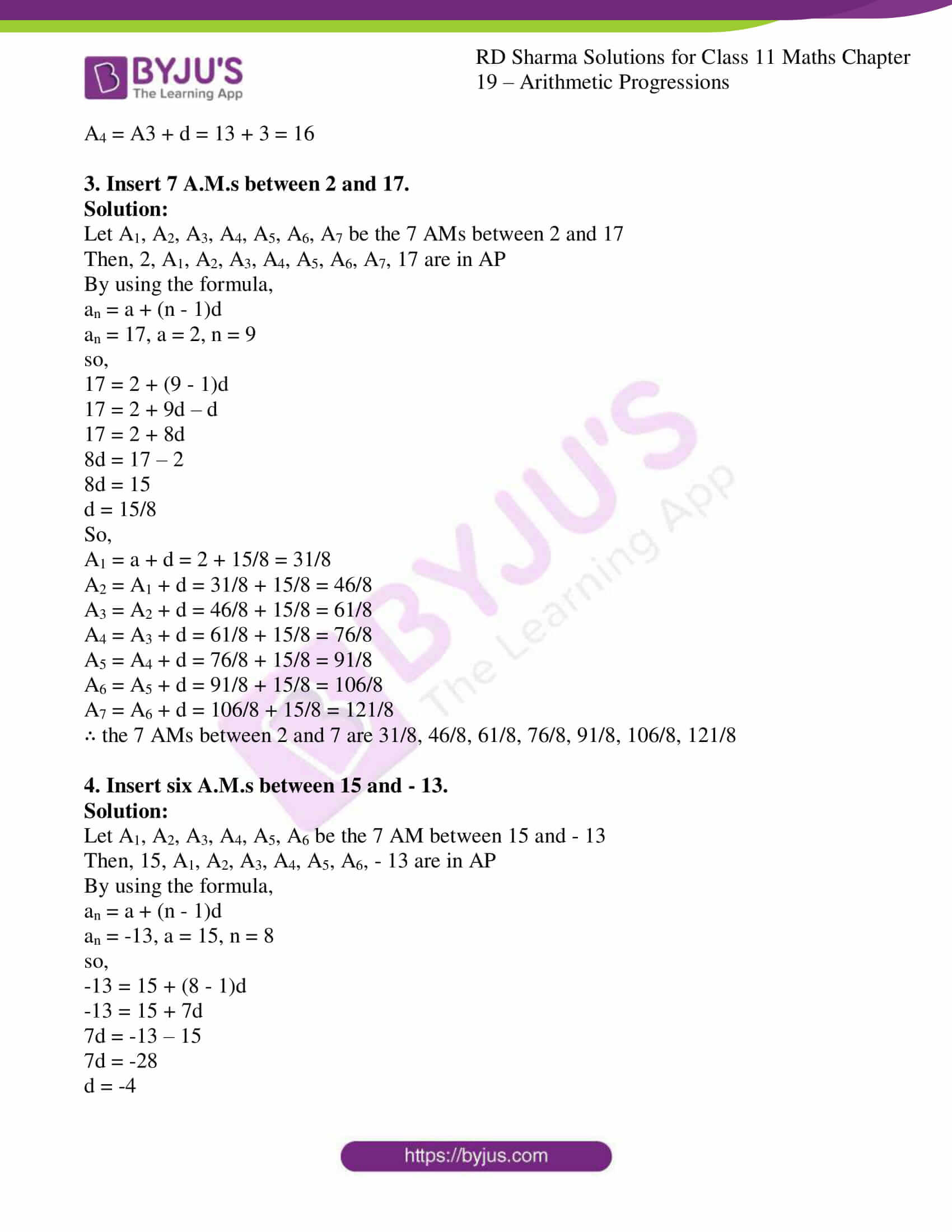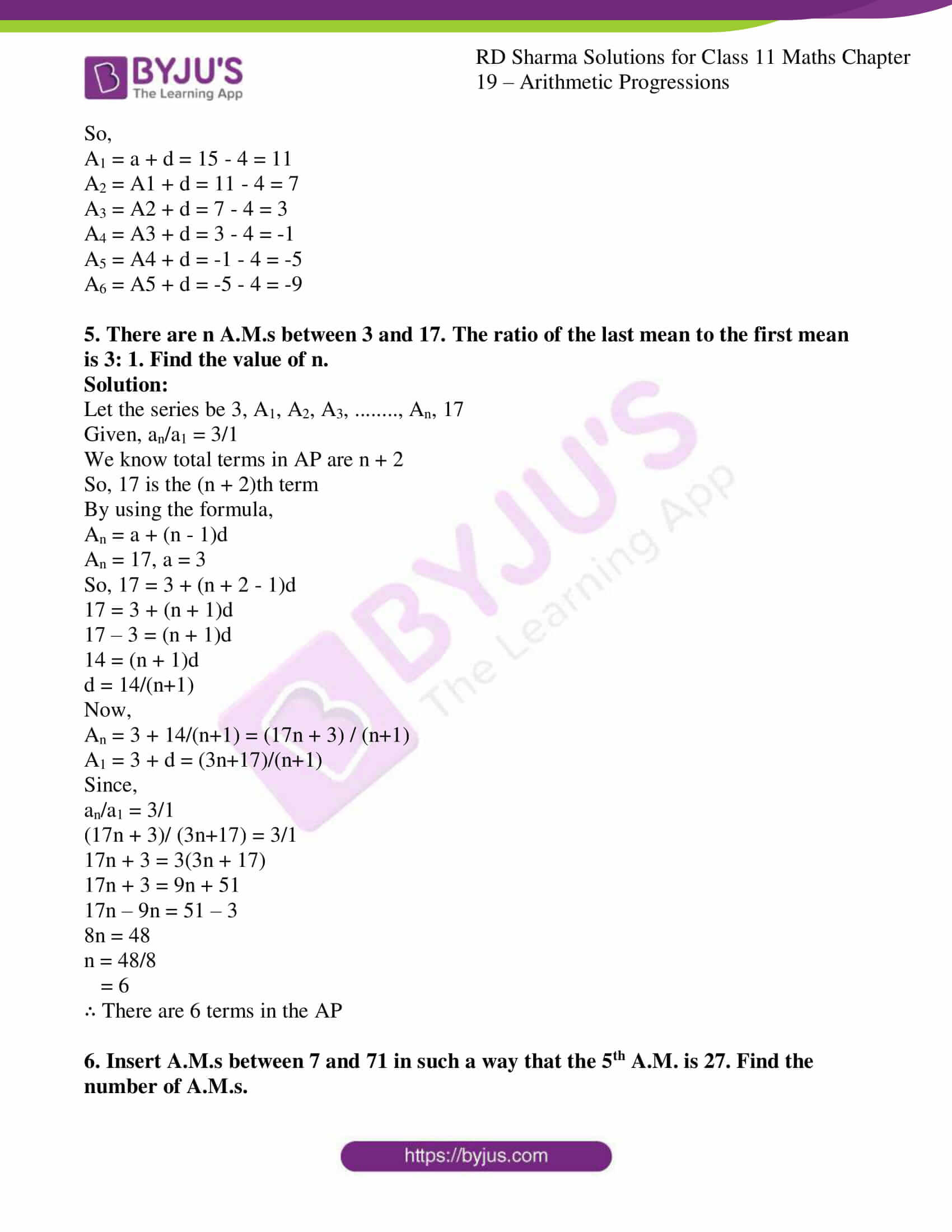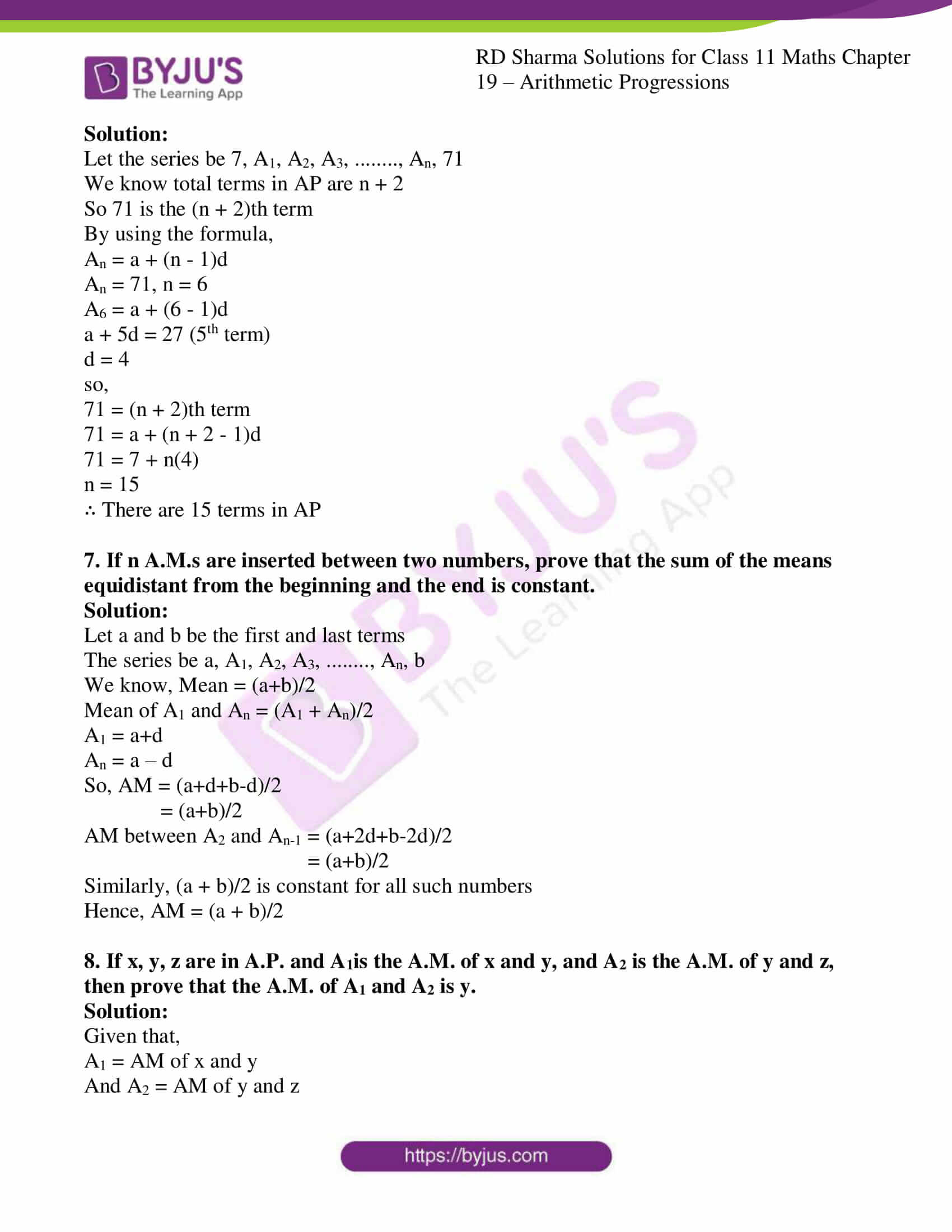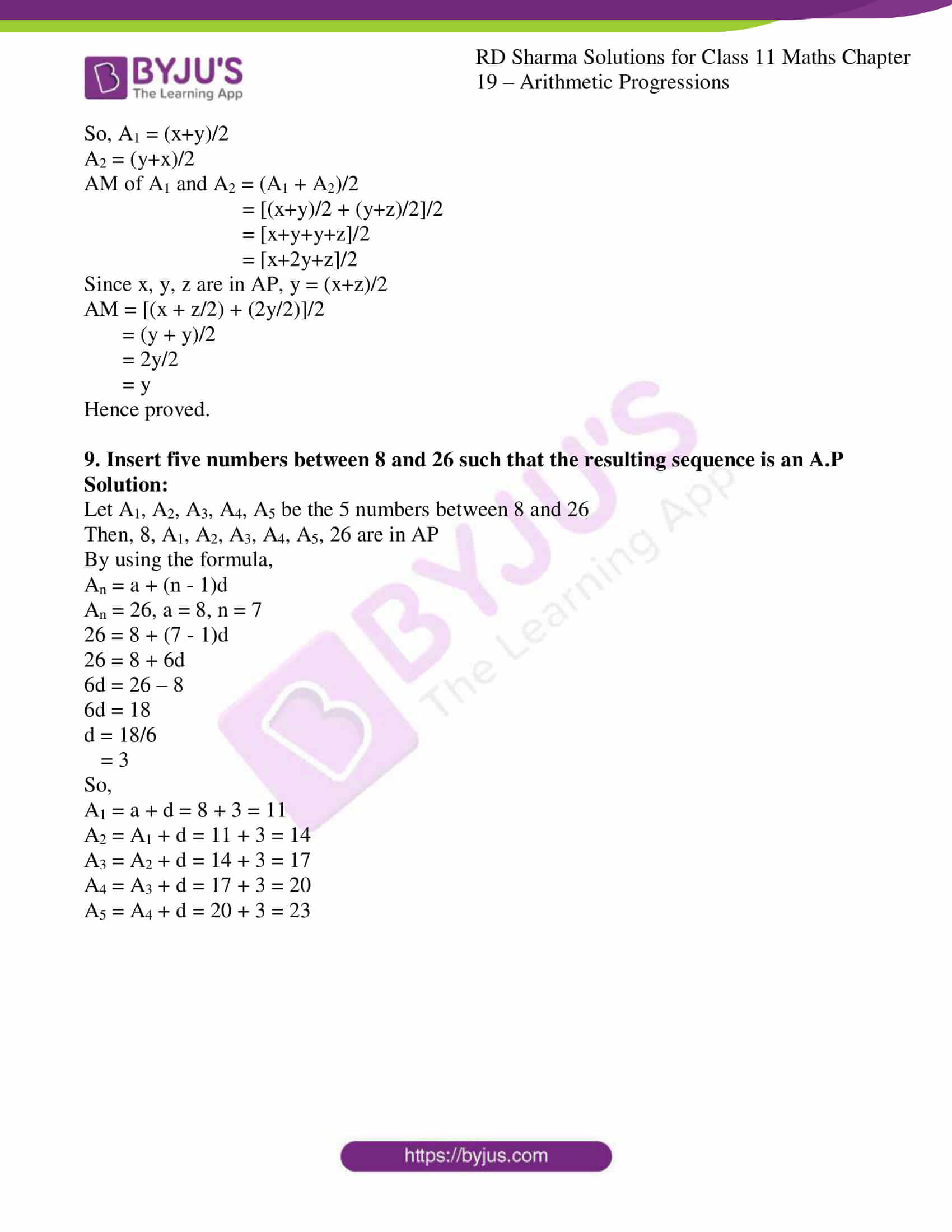### Also, access other exercises of RD Sharma Solutions for Class 11 Maths Chapter 19 – Arithmetic Progressions

Exercise 19.1 Solutions

Exercise 19.2 Solutions

Exercise 19.3 Solutions

Exercise 19.4 Solutions

Exercise 19.5 Solutions

Exercise 19.7 Solutions

### Access answers to RD Sharma Solutions for Class 11 Maths Exercise 19.6 Chapter 19 – Arithmetic Progressions

1. Find the A.M. between:
(i) 7 and 13 (ii) 12 and – 8 (iii) (x – y) and (x + y)

Solution:

(i) Let A be the Arithmetic mean

Then 7, A, 13 are in AP

Now, let us solve

A-7 = 13-A

2A = 13 + 7

A = 10

(ii) Let A be the Arithmetic mean

Then 12, A, – 8 are in AP

Now, let us solve

A – 12 = – 8 – A

2A = 12 + 8

A = 2

(iii) Let A be the Arithmetic mean

Then x – y, A, x + y are in AP

Now, let us solve

A – (x – y) = (x + y) – A

2A = x + y + x – y

A = x

2. Insert 4 A.M.s between 4 and 19.

Solution:

Let A1, A2, A3, A4 be the 4 AM Between 4 and 19

Then, 4, A1, A2, A3, A4, 19 are in AP.

By using the formula,

d = (b-a) / (n+1)

= (19 – 4) / (4 + 1)

= 15/5

= 3

So,

A1 = a + d = 4 + 3 = 7

A2 = A1 + d = 7 + 3 = 10

A3 = A2 + d = 10 + 3 = 13

A4 = A3 + d = 13 + 3 = 16

3. Insert 7 A.M.s between 2 and 17.

Solution:

Let A1, A2, A3, A4, A5, A6, A7 be the 7 AMs between 2 and 17

Then, 2, A1, A2, A3, A4, A5, A6, A7, 17 are in AP

By using the formula,

an = a + (n – 1)d

an = 17, a = 2, n = 9

so,

17 = 2 + (9 – 1)d

17 = 2 + 9d – d

17 = 2 + 8d

8d = 17 – 2

8d = 15

d = 15/8

So,

A1 = a + d = 2 + 15/8 = 31/8

A2 = A1 + d = 31/8 + 15/8 = 46/8

A3 = A2 + d = 46/8 + 15/8 = 61/8

A4 = A3 + d = 61/8 + 15/8 = 76/8

A5 = A4 + d = 76/8 + 15/8 = 91/8

A6 = A5 + d = 91/8 + 15/8 = 106/8

A7 = A6 + d = 106/8 + 15/8 = 121/8

∴ the 7 AMs between 2 and 7 are 31/8, 46/8, 61/8, 76/8, 91/8, 106/8, 121/8

4. Insert six A.M.s between 15 and – 13.

Solution:

Let A1, A2, A3, A4, A5, A6 be the 7 AM between 15 and – 13

Then, 15, A1, A2, A3, A4, A5, A6, – 13 are in AP

By using the formula,

an = a + (n – 1)d

an = -13, a = 15, n = 8

so,

-13 = 15 + (8 – 1)d

-13 = 15 + 7d

7d = -13 – 15

7d = -28

d = -4

So,

A1 = a + d = 15 – 4 = 11

A2 = A1 + d = 11 – 4 = 7

A3 = A2 + d = 7 – 4 = 3

A4 = A3 + d = 3 – 4 = -1

A5 = A4 + d = -1 – 4 = -5

A6 = A5 + d = -5 – 4 = -9

5. There are n A.M.s between 3 and 17. The ratio of the last mean to the first mean is 3: 1. Find the value of n.

Solution:

Let the series be 3, A1, A2, A3, …….., An, 17

Given, an/a1 = 3/1

We know total terms in AP are n + 2

So, 17 is the (n + 2)th term

By using the formula,

An = a + (n – 1)d

An = 17, a = 3

So, 17 = 3 + (n + 2 – 1)d

17 = 3 + (n + 1)d

17 – 3 = (n + 1)d

14 = (n + 1)d

d = 14/(n+1)

Now,

An = 3 + 14/(n+1) = (17n + 3) / (n+1)

A1 = 3 + d = (3n+17)/(n+1)

Since,

an/a1 = 3/1

(17n + 3)/ (3n+17) = 3/1

17n + 3 = 3(3n + 17)

17n + 3 = 9n + 51

17n – 9n = 51 – 3

8n = 48

n = 48/8

= 6

∴ There are 6 terms in the AP

6. Insert A.M.s between 7 and 71 in such a way that the 5th A.M. is 27. Find the number of A.M.s.

Solution:

Let the series be 7, A1, A2, A3, …….., An, 71

We know total terms in AP are n + 2

So 71 is the (n + 2)th term

By using the formula,

An = a + (n – 1)d

An = 71, n = 6

A6 = a + (6 – 1)d

a + 5d = 27 (5th term)

d = 4

so,

71 = (n + 2)th term

71 = a + (n + 2 – 1)d

71 = 7 + n(4)

n = 15

∴ There are 15 terms in AP

7. If n A.M.s are inserted between two numbers, prove that the sum of the means equidistant from the beginning and the end is constant.

Solution:

Let a and b be the first and last terms

The series be a, A1, A2, A3, …….., An, b

We know, Mean = (a+b)/2

Mean of A1 and An = (A1 + An)/2

A1 = a+d

An = a – d

So, AM = (a+d+b-d)/2

= (a+b)/2

AM between A2 and An-1 = (a+2d+b-2d)/2

= (a+b)/2

Similarly, (a + b)/2 is constant for all such numbers

Hence, AM = (a + b)/2

8. If x, y, z are in A.P. and A1is the A.M. of x and y, and A2 is the A.M. of y and z, then prove that the A.M. of A1 and A2 is y.

Solution:

Given that,

A1 = AM of x and y

And A2 = AM of y and z

So, A1 = (x+y)/2

A2 = (y+x)/2

AM of A1 and A2 = (A1 + A2)/2

= [(x+y)/2 + (y+z)/2]/2

= [x+y+y+z]/2

= [x+2y+z]/2

Since x, y, z are in AP, y = (x+z)/2

AM = [(x + z/2) + (2y/2)]/2

= (y + y)/2

= 2y/2

= y

Hence proved.

9. Insert five numbers between 8 and 26 such that the resulting sequence is an A.P

Solution:

Let A1, A2, A3, A4, A5 be the 5 numbers between 8 and 26

Then, 8, A1, A2, A3, A4, A5, 26 are in AP

By using the formula,

An = a + (n – 1)d

An = 26, a = 8, n = 7

26 = 8 + (7 – 1)d

26 = 8 + 6d

6d = 26 – 8

6d = 18

d = 18/6

= 3

So,

A1 = a + d = 8 + 3 = 11

A2 = A1 + d = 11 + 3 = 14

A3 = A2 + d = 14 + 3 = 17

A4 = A3 + d = 17 + 3 = 20

A5 = A4 + d = 20 + 3 = 23